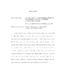## Commutative Endomorphism Rings of Simple Abelian Varieties over Finite FieldsIn this thesis we look at simple abelian varieties defined over a finite field $k =\mathbb{F}_{p^n}$ with $\End_k(A)$ commutative. We derive a formula that connects the $p$-rank $r(A)$ with the splitting behavior of $p$ in $E = \mathbb{Q}(\pi)$, where $\pi$ is a root of the characteristic polynomial of the Frobenius endomorphism. We show how this formula can be used to explicitly list all possible splitting behaviors of $p$ in $\mathcal{O}_E$, and we do so for abelian varieties of dimension less than or equal to four defined over $\mathbb{F}_p$. We then look for when $p$ divides $[\mathcal{O}_E : \mathbb{Z}[\pi, \bar{\pi}]]$. This allows us to prove that the endomorphism ring of an absolutely simple abelian surface is maximal at $p$ when $p \geq 3$. We also derive a condition that guarantees that $p$ divides $[\mathcal{O}_E: \mathbb{Z}[\pi, \bar{\pi}]]$. Last, we explicitly describe the structure of some intermediate subrings of $p$-power index between $\mathbb{Z}[\pi, \bar{\pi}]$ and $\mathcal{O}_E$ when $A$ is an abelian 3-fold with $r(A) = 1$.